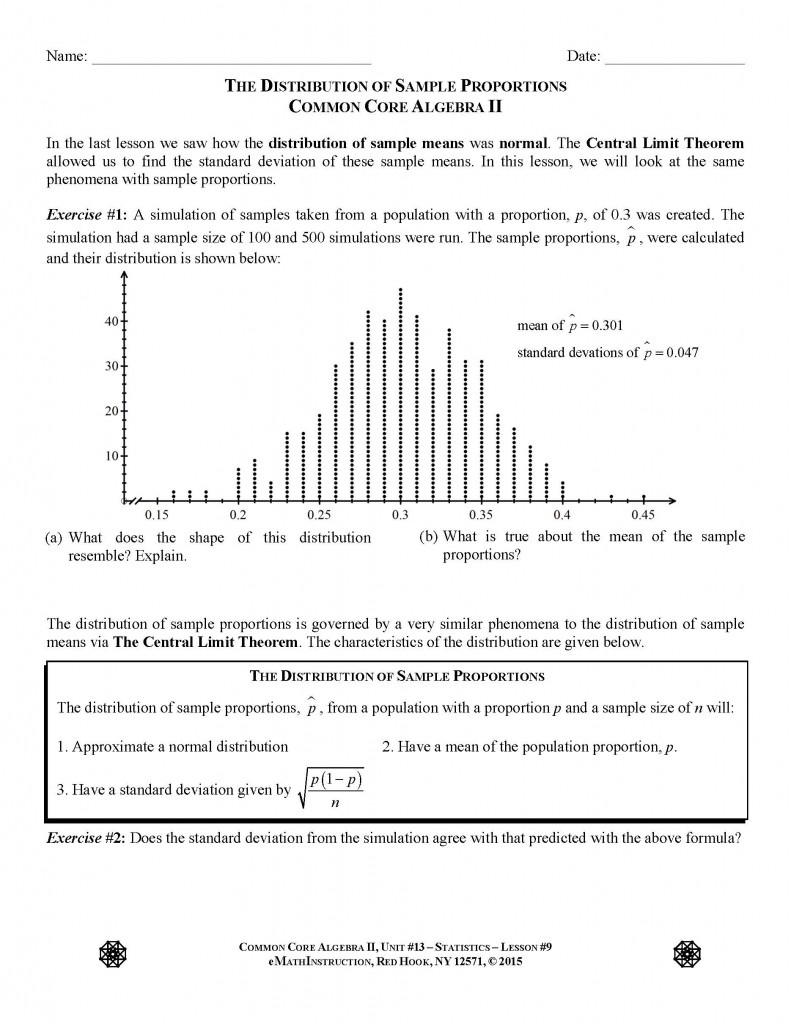Posted on

## New CC Algebra II Statistics Videos – by Kirk

A few weeks back, I published three new lessons to go with our final unit in Common Core Algebra II – Statistics. These lessons extended the work that we began in inferential statistics by introducing more formal ways of finding variation within sample statistics, such as sample means and proportions. By continuing to use simulation, we introduce students to the Central Limit Theorem for both sample means and sample proportions and extend this to a final lesson on margin of error. All of the lessons and their answer keys can be found at this post:

Simulation and Inferential Statistics – by Kirk

It took awhile, but I finally found time this weekend to record the videos for these lessons. I hope the videos are in time to help both students and teachers with this challenging material. I believe that these lessons will help students understand inferential statistics and how the results of the simulations can be extended to standard methods within statistics. Here are the YouTube links to the three new videos:

CC Alg II.Unit #13.Lesson #8.The Distribution of Sample Means

CC Alg II.Unit #13.Lesson #9.The Distribution of Sample Proportions

CC Alg II.Unit #13.Lesson #10.Margin of Error

I know that the numbering system here is in conflict with our final two lessons on regression. We will be re-numbering those last two lessons on regression as Lessons #11 and #12 respectively (as well as figuring out where they really make sense in the overall text).

Posted on

## Statistical Simulators and Additional Lessons – Common Core Algebra II

Well, it’s getting to be crunch time in Common Core Algebra II now that April looms before us. As at Arlington, I’m sure that many districts are beginning Probability soon and will then turn to statistics in Common Core Algebra II.

I still am a little in the dark about the expectations that the state has in terms of what it wants students to know and be able to do when it comes to inferential statistics. Some of what we know comes from the CC Standards themselves, some comes from the Modules, and some comes from the sample questions. But, these three things are often contradictory.

At the end of the day, I feel like the original curriculum that I created does a good job at using Statistical Simulation to give students a good feel for how probability ties into inferential statistics. I’ve written statistical simulators for the TI-83/84 calculators and much, much faster simulators that are web-based. I describe all of these simulators in detail and supply links to the calculator code and web based apps in this earlier post of mine:

Statistical Simulation in Common Core Algebra II

I decided to add three additional lessons to the inferential statistics curriculum and am offering them up here in their pdf form. The answer keys will be available on the new answer key for CC Algebra II but aren’t available now. These three lessons take a more classic, formula based approach to inferential statistics, particularly margin of error for both means and proportions. The lessons are numbered a bit strangely because they will fit after all of the other simulation lessons but before our final two lesson on regression. Here they are:

CCAlgII.Unit #13.Lesson #8.The Distribution of Sample Means

CCAlgII.Unit #13.Lesson #9.The Distribution of Sample Proportions

CCAlgII.Unit #13.Lesson #10.Margin of Error